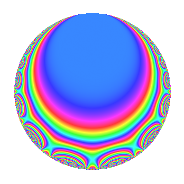# Properties

 Label 3600.1.bbLevel $3600$ Weight $1$ Character orbit 3600.bb Rep. character $\chi_{3600}(757,\cdot)$ Character field $\Q(\zeta_{4})$ Dimension $4$ Newform subspaces $2$ Sturm bound $720$ Trace bound $17$

# Related objects

## Defining parameters

 Level: $$N$$ $$=$$ $$3600 = 2^{4} \cdot 3^{2} \cdot 5^{2}$$ Weight: $$k$$ $$=$$ $$1$$ Character orbit: $$[\chi]$$ $$=$$ 3600.bb (of order $$4$$ and degree $$2$$) Character conductor: $$\operatorname{cond}(\chi)$$ $$=$$ $$80$$ Character field: $$\Q(i)$$ Newform subspaces: $$2$$ Sturm bound: $$720$$ Trace bound: $$17$$

## Dimensions

The following table gives the dimensions of various subspaces of $$M_{1}(3600, [\chi])$$.

Total New Old
Modular forms 52 8 44
Cusp forms 4 4 0
Eisenstein series 48 4 44

The following table gives the dimensions of subspaces with specified projective image type.

$$D_n$$ $$A_4$$ $$S_4$$ $$A_5$$
Dimension 4 0 0 0

## Trace form

 $$4q - 4q^{4} + O(q^{10})$$ $$4q - 4q^{4} + 4q^{16} - 4q^{19} + 4q^{34} + 4q^{46} + 4q^{61} - 4q^{64} + 4q^{76} + 4q^{94} + O(q^{100})$$

## Decomposition of $$S_{1}^{\mathrm{new}}(3600, [\chi])$$ into newform subspaces

Label Dim. $$A$$ Field Image CM RM Traces $q$-expansion
$$a_2$$ $$a_3$$ $$a_5$$ $$a_7$$
3600.1.bb.a $$2$$ $$1.797$$ $$\Q(\sqrt{-1})$$ $$D_{4}$$ $$\Q(\sqrt{-15})$$ None $$0$$ $$0$$ $$0$$ $$0$$ $$q-iq^{2}-q^{4}+iq^{8}+q^{16}+(-1+i+\cdots)q^{17}+\cdots$$
3600.1.bb.b $$2$$ $$1.797$$ $$\Q(\sqrt{-1})$$ $$D_{4}$$ $$\Q(\sqrt{-15})$$ None $$0$$ $$0$$ $$0$$ $$0$$ $$q+iq^{2}-q^{4}-iq^{8}+q^{16}+(1-i+\cdots)q^{17}+\cdots$$

## Hecke characteristic polynomials

$p$ $F_p(T)$
$2$ ($$1 + T^{2}$$)($$1 + T^{2}$$)
$3$ 1
$5$ 1
$7$ ($$1 + T^{4}$$)($$1 + T^{4}$$)
$11$ ($$1 + T^{4}$$)($$1 + T^{4}$$)
$13$ ($$( 1 - T )^{2}( 1 + T )^{2}$$)($$( 1 - T )^{2}( 1 + T )^{2}$$)
$17$ ($$( 1 + T )^{2}( 1 + T^{2} )$$)($$( 1 - T )^{2}( 1 + T^{2} )$$)
$19$ ($$( 1 + T )^{2}( 1 + T^{2} )$$)($$( 1 + T )^{2}( 1 + T^{2} )$$)
$23$ ($$( 1 + T )^{2}( 1 + T^{2} )$$)($$( 1 - T )^{2}( 1 + T^{2} )$$)
$29$ ($$1 + T^{4}$$)($$1 + T^{4}$$)
$31$ ($$( 1 + T^{2} )^{2}$$)($$( 1 + T^{2} )^{2}$$)
$37$ ($$( 1 - T )^{2}( 1 + T )^{2}$$)($$( 1 - T )^{2}( 1 + T )^{2}$$)
$41$ ($$( 1 - T )^{2}( 1 + T )^{2}$$)($$( 1 - T )^{2}( 1 + T )^{2}$$)
$43$ ($$( 1 + T^{2} )^{2}$$)($$( 1 + T^{2} )^{2}$$)
$47$ ($$( 1 + T )^{2}( 1 + T^{2} )$$)($$( 1 - T )^{2}( 1 + T^{2} )$$)
$53$ ($$( 1 + T )^{4}$$)($$( 1 - T )^{4}$$)
$59$ ($$1 + T^{4}$$)($$1 + T^{4}$$)
$61$ ($$( 1 - T )^{2}( 1 + T^{2} )$$)($$( 1 - T )^{2}( 1 + T^{2} )$$)
$67$ ($$( 1 + T^{2} )^{2}$$)($$( 1 + T^{2} )^{2}$$)
$71$ ($$( 1 - T )^{2}( 1 + T )^{2}$$)($$( 1 - T )^{2}( 1 + T )^{2}$$)
$73$ ($$1 + T^{4}$$)($$1 + T^{4}$$)
$79$ ($$( 1 + T^{2} )^{2}$$)($$( 1 + T^{2} )^{2}$$)
$83$ ($$( 1 + T^{2} )^{2}$$)($$( 1 + T^{2} )^{2}$$)
$89$ ($$( 1 + T^{2} )^{2}$$)($$( 1 + T^{2} )^{2}$$)
$97$ ($$1 + T^{4}$$)($$1 + T^{4}$$)